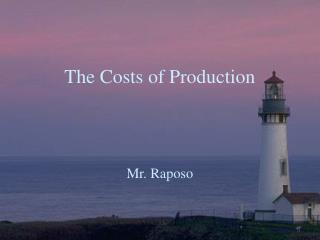# The Costs of Production - PowerPoint PPT PresentationDownload PresentationThe Costs of Production

The Costs of ProductionDownload Presentation## The Costs of Production

- - - - - - - - - - - - - - - - - - - - - - - - - - - E N D - - - - - - - - - - - - - - - - - - - - - - - - - - -
##### Presentation Transcript

1. The Costs of Production Mr. Raposo

2. What is a business? • Business: An enterprise that brings individuals, financial and economic resources to produce goods or • services. • Sole Proprietorship: A business owned by a single person. • Partnership: A business owned by two or more people. • Corporation: A company given legal status apart from its owners. Ownership in a corporation is gained (loss) by buying (selling) shares.

3. What is Production? • Production: The process of transforming our resources (inputs) into a good or service with economic value (output). • Inputs: Resources used in production • Output: The quantity of a good or service that results from production

4. Labour Intensive vs. Capital Intensive Processes • Labour-intensive process: Employs more labour less capital in its production process • Capital-intensive process: Employs more capital and less labour in its production process.

5. Example of Productive Efficiencyfig. 4.2 Labour Intensive Capital Intensive

6. Productive Efficiency • Productive Efficiency: Producing a certain quantity of output at the lowest cost. • Example: Worker in previous example costs \$100/day. The cost of the machine is \$25 day. Which production process is more efficient?

7. Exercise: Measure Productive Efficiency

9. Economic Costs vs. Accounting Costs • Economic Costs: Include both Explicit and Implicit costs • Explicit Costs: Expenses such as payment for material, wages, rent, etc. These costs appear on a business firm's accounting records. • Implicit Costs: These costs not included among expenses on the accounting records of a business firm. They are estimates of what the owner’s “give up” by being involved in the business.

10. Economic Profit Economic Profit = Total revenue – economic costs Remember, Total Revenue (TR) = Price (P) x Quantity(Q)

11. Accounting Profit • Excludes implicit costs • Accounting Profit = Total revenue-Explicit Costs

12. Example: • Widget company has total revenue of \$200,000. Accountants determine explicit costs to be \$150,000. The owner, Mr. R, of widget co. has invested \$80,000 of his own money that could earn 10% on \$80,000 if invested. Mr. R could also be earning at least \$45,000 as a manager at his previous work. Calculate accounting and economic profit.

13. Exercise • Calculate Accounting and Economic Profit

14. Example: • Widget company has total revenue of \$200,000. Accountants determine explicit costs to be \$150,000. The owner, Mr. R, of widget co. has invested \$80,000 of his own money that could earn 10% on \$80,000 if invested. Mr. R could also be earning at least \$45,000 as a manager at his previous work. Calculate accounting and economic profit.

15. Accounting Profit = Total Revenue – Explicit Costs = \$200,000 - \$150,000 = \$50,000

16. Economic Profit = Total Revenue – Economic Costs = Total Revenue – (Explicit + Implicit Costs) = \$200,000 – (\$150,000+\$8,000+\$45,000) = \$200,000 – \$203,000 = -\$3,000 (a loss) (Interest Calculated as: \$80,000 x 10% = \$8,000)

17. Homework: p. 121#1-5

18. Production in the Short-Run • Short-run: A period of time too short for a business to alter its plant capacity, but long enough to vary the degree to which this capacity is utilized. • Long-run: A period of time long enough to permit a firm to vary the capacity of the plant as well as the degree of its use.

19. Using Resources • Fixed Inputs: Inputs that cannot be adjusted in the short run. (ex: Size of machine or factory) • Variable Inputs: Inputs that can be adjusted in the short run. (ex: labour and materials)

20. Total, Average and Marginal Product • Total product = q (quantity of output) • Average product = total product (q) (AP) # of workers (L) • Marginal product = q L

21. Calculate and Graph • Calculate and total, marginal and average product • Graph total product on a separate graph • Graph marginal and average product together on a separate graph below the total product graph • Refer to text fig.4.4 p. 106

22. Fig. 4.3 Production in the Short Run

23. Fig. 4.3 Production in the Short Run

24. The Costs of Production • Fixed, Variable & Total Costs • Per Unit Costs (or Average Costs) • Marginal Costs

25. Law of Diminishing Marginal Returns • Law of diminishing marginal returns: At a certain point, the addition of a variable input to a fixed input causes the marginal product to decrease.

26. Law of Diminishing Marginal Returns • Example: Class activity for Mr. R’s widget co.

27. Short Run & Long Run • Short Run- a period in which at least one of a firm’s resources is fixed (plant capacity) • Output can be varied by increasing labour, materials & other resources but plant capacity is fixed • Long Run- a period in which all resources are variable, including plant capacity

28. Fixed, Variable & Total Costs • Fixed Costs = Costs that do not vary with output • Variable Costs = Costs that vary with output • Total Costs = Fixed + Variable Costs

29. Per Unit or Average Costs • Average Fixed Costs (AFC) = TFC Q • Average Variable Costs (AVC) = TVC Q • Average Total Costs (ATC) = TFC+TVC Q

30. Marginal Cost • Marginal Cost (MC): the additional cost of producing one more unit of output. • Marginal Cost = Change in Total Cost Change in Q

31. Application • Calculate and graph marginal cost, average cost, average variable costs and average fixed costs • Refer to text p. 111

32. Short-run costs: Enter formula in first row